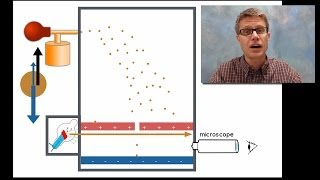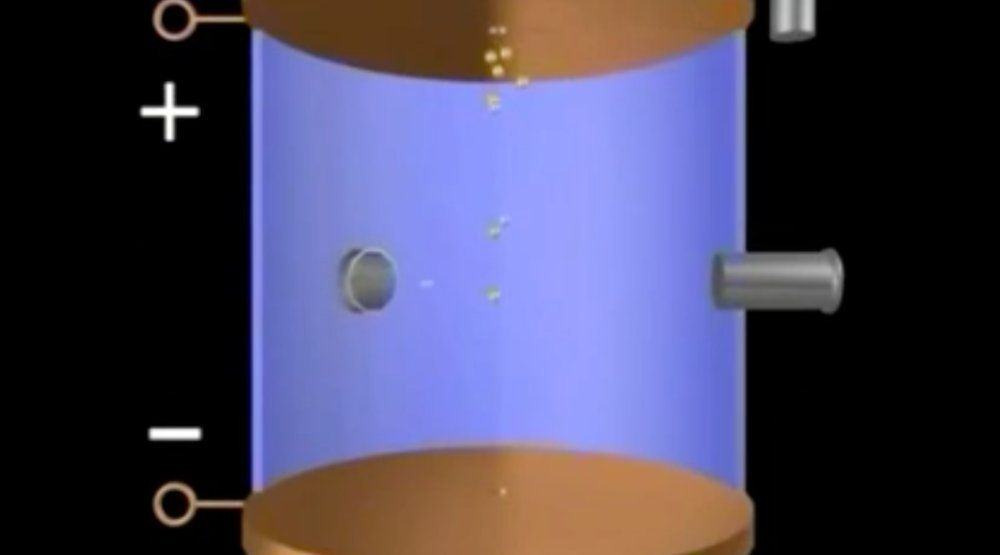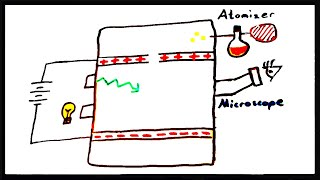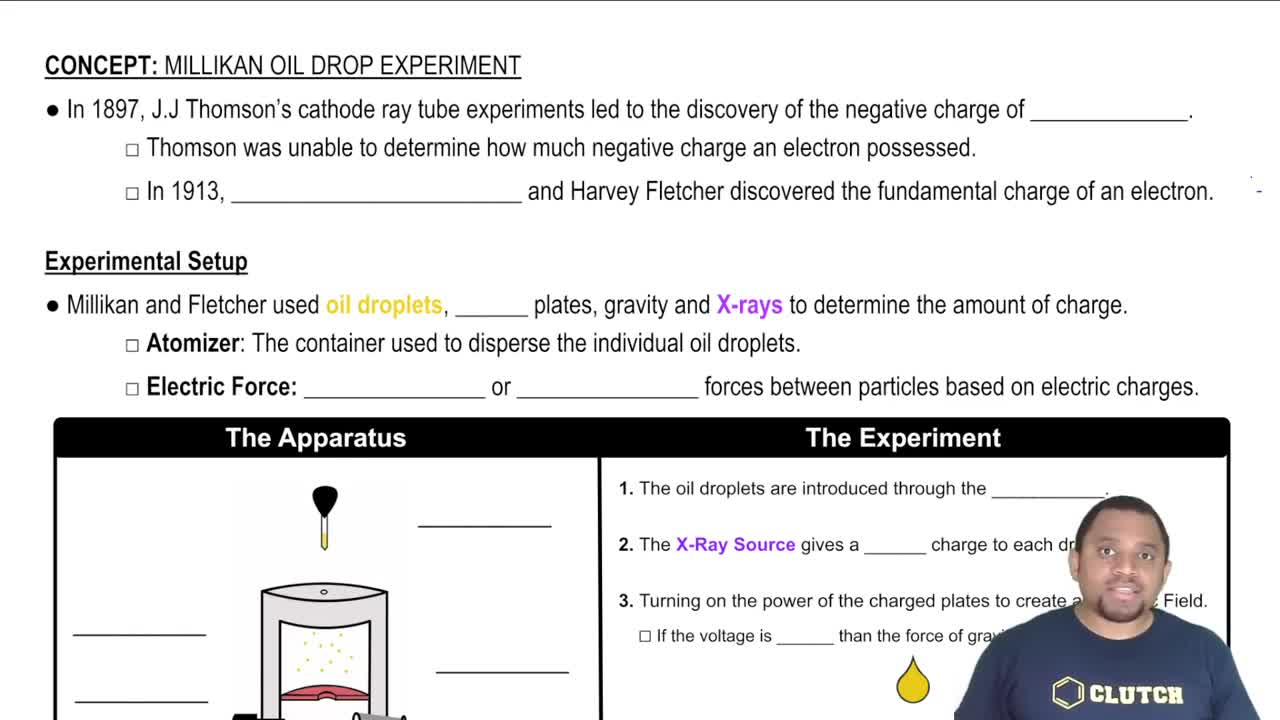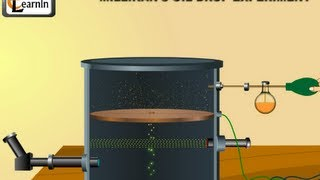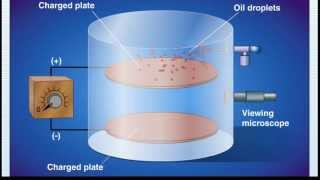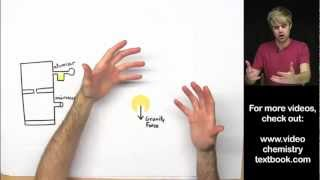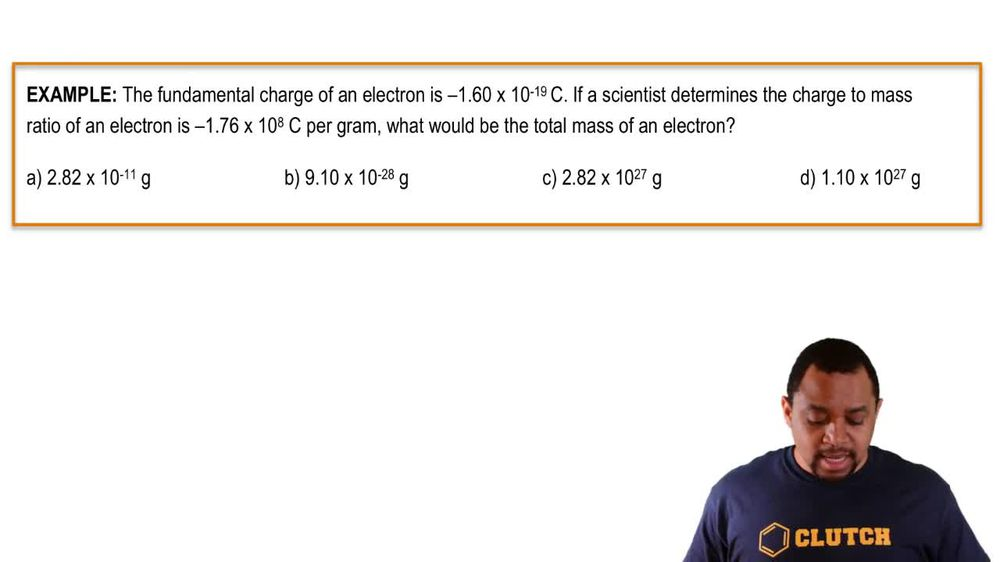Start typing, then use the up and down arrows to select an option from the list.
1. 2. Atoms & Elements2. Millikan Oil Drop Experiment
Problem

# Which of the following charges is not possible for the over-all charge on an oil droplet in Millikan’s experiment? For this problem, we’ll round the currently accepted charge of an electron to 1.602 * 10-19 C. (a) -1.010 * 10-18 C (b) -8.010 * 10-19 C (c) -2.403 * 10-18 C

Relevant Solution2m
Play a video:
Hello everyone today. We have been given the following problem identify the unlikely charge of an oil droplet. In Millikan experiment use E. Is equal to 1.602, 2 times 10 to the 19th charge or columns for the charge of an electron. So essentially we want to identify an electric charge that is essentially not an integer. So that is not an integer when we divide by this value. So for a we are going to take this 6.4088 times 10 to the negative 19th columns and divide that by our value of E. And we actually get four And four is an integer. Therefore answer choice A cannot be correct. And we're gonna go ahead and repeat this process for each of these answer choices. So when we calculate this for B we get seven which is also an integer. Moving on to see we have 5.3407 times 10 to the negative 18th columns. We divide that by E 1.6022 times 10 to the negative 19th columns. And we actually get 1/3 which is not an integer, which is actually going to be our answer. And so with that and I see is our answer. Ultimately, I hope this helped. And until next time